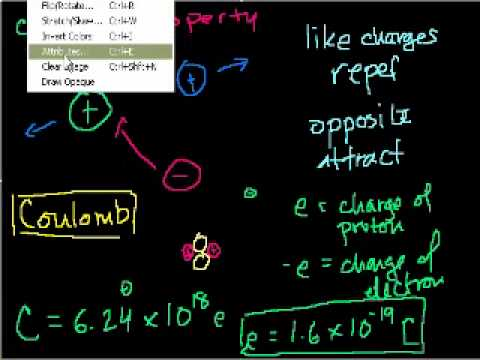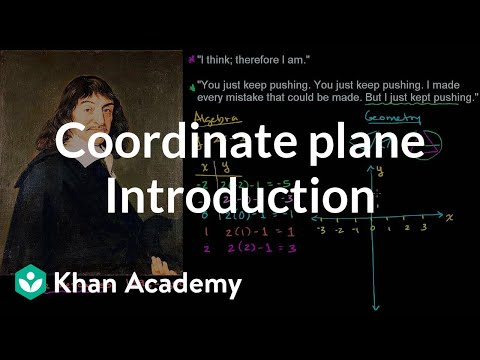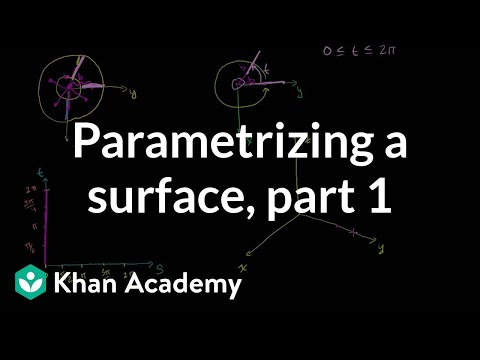#### educationproviderprofile

The Khan Academy is an organization on a mission. We're a not-for-profit with the goal of changing education for the better by providing a free world-class education for anyone anywhere. All of the site's resources are available to anyone. It doesn't matter if you are a student, teacher, home-schooler, principal, adult returning to the classroom after 20 years, or a friendly alien just trying to get a leg up in earthly biology. The Khan Academy's materials and resources are available to you completely free of charge.
View reviews or write your own
Find alternative courses to courses from Khan Academy
Find exams to get certification or college credits after courses from Khan Academy
See infigraphic and subjects clouds

# News

### Remote learning with Khan Academy during school closures

2020-03-05 17:32:59

Resources for schools, teachers, and parentsHello teachers and parents,We know many schools and families are making plans to be prepared sho…

2020-03-02 17:04:49

Khan Academy Kids, our award-winning educational app for children ages two to seven, now includes new first-grade lessons. We’ve added…

2020-02-18 18:17:29

At Khan Academy we love to learn. As a learning organization, we often share book recommendations with one another. In honor of Black Histor…

### College Board & Khan Academy Mark 10 Million Milestone of Free Official SAT® Practice

2020-01-27 19:42:49

More than 10 million students have signed up for free SAT practice on Khan Academy in 5 yearsIn 2015, College Board and Khan Academy partner…

### 20 educational gifts for all ages: Khan Academy team’s favorite toys, books, and games for learning

2019-12-11 16:27:55

It’s the season of giving! We asked the Khan Academy content team to share the gifts they love to give the children in their lives. Fi…

# Top courses Most popular

### Applied math: Ancient CryptographyProvider: Khan AcademyExplore how we have hidden secret messages through history. What is Cryptography?. Probability Space. The Caesar Cipher. Polyalphabetic Cipher. The One-Time Pad. Frequency Stability. The Enigma Encryption Machine (case study). Perfect Secrecy. Pseudorandom Number Generators.

### Physics: Electricity and magnetismProvider: Khan AcademyElectrostatics (part 1): Introduction to Charge and Coulomb's Law. Electrostatics (part 2). Proof (Advanced): Field from infinite plate (part 1). Proof (Advanced): Field from infinite plate (part 2). Electric Potential Energy. Electric Potential Energy (part 2-- involves calculus). Voltage. Capacitance. Circuits (part 1). Circuits (part 2). Circuits (part 3). Circuits (part 4). Cross product 1. Cross Product 2. Cross Product and Torque. Introduction to Magnetism. Magnetism 2. Magnetism 3. Magnetism 4. Magnetism 5. Magnetism 6: Magnetic field due to current. Magnetism 7. Magnetism 8. Magnetism 9: Electric Motors. Magnetism 10: Electric Motors. Magnetism 11: Electric Motors. Magnetism 12: Induced Current in a Wire. The dot product. Dot vs. Cross Product. Calculating dot and cross products with unit vector notation. Electrostatics (part 1): Introduction to Charge and Coulomb's Law. Electrostatics (part 2). Proof (Advanced): Field from infinite plate (part 1). Proof (Advanced): Field from infinite plate (part 2). Electric Potential Energy. Electric Potential Energy (part 2-- involves calculus). Voltage. Capacitance. Circuits (part 1). Circuits (part 2). Circuits (part 3). Circuits (part 4). Cross product 1. Cross Product 2. Cross Product and Torque. Introduction to Magnetism. Magnetism 2. Magnetism 3. Magnetism 4. Magnetism 5. Magnetism 6: Magnetic field due to current. Magnetism 7. Magnetism 8. Magnetism 9: Electric Motors. Magnetism 10: Electric Motors. Magnetism 11: Electric Motors. Magnetism 12: Induced Current in a Wire. The dot product. Dot vs. Cross Product. Calculating dot and cross products with unit vector notation.

### Algebra: Graphing and analyzing linear functionsProvider: Khan AcademyUse the power of algebra to understand and interpret points and lines (something we typically do in geometry). This will include slope and the equation of a line. Descartes and Cartesian Coordinates. The Coordinate Plane. Plot ordered pairs. Graphing points exercise. Graphing points. Quadrants of Coordinate Plane. Graphing points and naming quadrants exercise. Graphing points and naming quadrants. Points on the coordinate plane. Points on the coordinate plane. Coordinate plane word problems exercise. Coordinate plane word problems. Reflecting points exercise. Reflecting points. Ordered pair solutions of equations. Ordered Pair Solutions of Equations 2. Determining a linear equation by trying out values from a table. Equations from tables. Plotting (x,y) relationships. Graphs of Linear Equations. Application problem with graph. Ordered pair solutions to linear equations. Interpreting Linear Graphs. Exploring linear relationships. Recognizing Linear Functions. Interpreting linear relationships. Graphing lines 1. Recognizing Linear Functions. Linear and nonlinear functions (example 1). Linear and nonlinear functions (example 2). Linear and nonlinear functions (example 3). Linear and nonlinear functions. Graphing using X and Y intercepts. Graphing Using Intercepts. X and Y intercepts. X and Y intercepts 2. Solving for the x-intercept. Finding x intercept of a line. Finding intercepts for a linear function from a table. Linear function intercepts. Interpreting intercepts of linear functions. Interpreting and finding intercepts of linear functions. Analyzing and identifying proportional relationships ex1. Analyzing and identifying proportional relationships ex2. Analyzing and identifying proportional relationships ex3. Analyzing and identifying proportional relationships. Comparing proportional relationships. Constructing an equation for a proportional relationship. Constructing and comparing proportional relationships. Graphing proportional relationships example. Graphing proportional relationships example 2. Graphing proportional relationships example 3. Graphing proportional relationships. Comparing rates. Representing and comparing rates. Rates and proportional relationships. Rate problem with fractions 1. Unit cost with fractions 1. Rate problems 1. Slope of a line. Slope of a Line 2. Slope and Rate of Change. Graphical Slope of a Line. Slope of a Line 3. Slope Example. Hairier Slope of Line. Identifying slope of a line. Slope and Y-intercept Intuition. Line graph intuition. Algebra: Slope. Algebra: Slope 2. Algebra: Slope 3. Graphing a line in slope intercept form. Converting to slope-intercept form. Graphing linear equations. Fitting a Line to Data. Comparing linear functions 1. Comparing linear functions 2. Comparing linear functions 3. Comparing linear functions. Interpreting features of linear functions example. Interpreting features of linear functions example 2. Interpreting features of linear functions. Comparing linear functions applications 1. Comparing linear functions applications 2. Comparing linear functions applications 3. Comparing linear functions applications. Constructing a linear function word problem. Constructing and interpreting a linear function. Constructing linear graphs. Constructing and interpreting linear functions. Multiple examples of constructing linear equations in slope-intercept form. Constructing equations in slope-intercept form from graphs. Constructing linear equations to solve word problems. Linear equation from slope and a point. Finding a linear equation given a point and slope. Equation of a line from fractional slope and point. Constructing the equation of a line given two points. Finding y intercept given slope and point. Solving for the y-intercept. Slope intercept form from table. Slope intercept form. Idea behind point slope form. Linear Equations in Point Slope Form. Point slope form. Linear Equations in Standard Form. Point-slope and standard form. Converting between slope-intercept and standard form. Converting from point slope to slope intercept form. Converting between point-slope and slope-intercept. Finding the equation of a line. Midpoint formula. Midpoint formula. The Pythagorean theorem intro. Pythagorean theorem. Distance Formula. Distance formula. Perpendicular Line Slope. Equations of Parallel and Perpendicular Lines. Parallel Line Equation. Parallel Lines. Parallel Lines 2. Parallel lines 3. Perpendicular Lines. Perpendicular lines 2. Equations of parallel and perpendicular lines. Distance between a point and a line. Distance between point and line. Algebra: Slope and Y-intercept intuition. Algebra: Equation of a line. CA Algebra I: Slope and Y-intercept. Graphing Inequalities. Solving and graphing linear inequalities in two variables 1. Graphing Linear Inequalities in Two Variables Example 2. Graphing Inequalities 2. Graphing linear inequalities in two variables 3. Graphs of inequalities. Graphing linear inequalities. Graphing Inequalities 1. Graphing and solving linear inequalities. CA Algebra I: Graphing Inequalities. Similar triangles to prove that the slope is constant for a line. Slope and triangle similarity 1. Slope and triangle similarity 2. Slope and triangle similarity 3. Slope and triangle similarity 4. Slope and triangle similarity. Average Rate of Change Example 1). Average Rate of Change Example 2). Average Rate of Change Example 3). Average rate of change when function defined by equation. Average rate of change. Descartes and Cartesian Coordinates. The Coordinate Plane. Plot ordered pairs. Graphing points exercise. Graphing points. Quadrants of Coordinate Plane. Graphing points and naming quadrants exercise. Graphing points and naming quadrants. Points on the coordinate plane. Points on the coordinate plane. Coordinate plane word problems exercise. Coordinate plane word problems. Reflecting points exercise. Reflecting points. Ordered pair solutions of equations. Ordered Pair Solutions of Equations 2. Determining a linear equation by trying out values from a table. Equations from tables. Plotting (x,y) relationships. Graphs of Linear Equations. Application problem with graph. Ordered pair solutions to linear equations. Interpreting Linear Graphs. Exploring linear relationships. Recognizing Linear Functions. Interpreting linear relationships. Graphing lines 1. Recognizing Linear Functions. Linear and nonlinear functions (example 1). Linear and nonlinear functions (example 2). Linear and nonlinear functions (example 3). Linear and nonlinear functions. Graphing using X and Y intercepts. Graphing Using Intercepts. X and Y intercepts. X and Y intercepts 2. Solving for the x-intercept. Finding x intercept of a line. Finding intercepts for a linear function from a table. Linear function intercepts. Interpreting intercepts of linear functions. Interpreting and finding intercepts of linear functions. Analyzing and identifying proportional relationships ex1. Analyzing and identifying proportional relationships ex2. Analyzing and identifying proportional relationships ex3. Analyzing and identifying proportional relationships. Comparing proportional relationships. Constructing an equation for a proportional relationship. Constructing and comparing proportional relationships. Graphing proportional relationships example. Graphing proportional relationships example 2. Graphing proportional relationships example 3. Graphing proportional relationships. Comparing rates. Representing and comparing rates. Rates and proportional relationships. Rate problem with fractions 1. Unit cost with fractions 1. Rate problems 1. Slope of a line. Slope of a Line 2. Slope and Rate of Change. Graphical Slope of a Line. Slope of a Line 3. Slope Example. Hairier Slope of Line. Identifying slope of a line. Slope and Y-intercept Intuition. Line graph intuition. Algebra: Slope. Algebra: Slope 2. Algebra: Slope 3. Graphing a line in slope intercept form. Converting to slope-intercept form. Graphing linear equations. Fitting a Line to Data. Comparing linear functions 1. Comparing linear functions 2. Comparing linear functions 3. Comparing linear functions. Interpreting features of linear functions example. Interpreting features of linear functions example 2. Interpreting features of linear functions. Comparing linear functions applications 1. Comparing linear functions applications 2. Comparing linear functions applications 3. Comparing linear functions applications. Constructing a linear function word problem. Constructing and interpreting a linear function. Constructing linear graphs. Constructing and interpreting linear functions. Multiple examples of constructing linear equations in slope-intercept form. Constructing equations in slope-intercept form from graphs. Constructing linear equations to solve word problems. Linear equation from slope and a point. Finding a linear equation given a point and slope. Equation of a line from fractional slope and point. Constructing the equation of a line given two points. Finding y intercept given slope and point. Solving for the y-intercept. Slope intercept form from table. Slope intercept form. Idea behind point slope form. Linear Equations in Point Slope Form. Point slope form. Linear Equations in Standard Form. Point-slope and standard form. Converting between slope-intercept and standard form. Converting from point slope to slope intercept form. Converting between point-slope and slope-intercept. Finding the equation of a line. Midpoint formula. Midpoint formula. The Pythagorean theorem intro. Pythagorean theorem. Distance Formula. Distance formula. Perpendicular Line Slope. Equations of Parallel and Perpendicular Lines. Parallel Line Equation. Parallel Lines. Parallel Lines 2. Parallel lines 3. Perpendicular Lines. Perpendicular lines 2. Equations of parallel and perpendicular lines. Distance between a point and a line. Distance between point and line. Algebra: Slope and Y-intercept intuition. Algebra: Equation of a line. CA Algebra I: Slope and Y-intercept. Graphing Inequalities. Solving and graphing linear inequalities in two variables 1. Graphing Linear Inequalities in Two Variables Example 2. Graphing Inequalities 2. Graphing linear inequalities in two variables 3. Graphs of inequalities. Graphing linear inequalities. Graphing Inequalities 1. Graphing and solving linear inequalities. CA Algebra I: Graphing Inequalities. Similar triangles to prove that the slope is constant for a line. Slope and triangle similarity 1. Slope and triangle similarity 2. Slope and triangle similarity 3. Slope and triangle similarity 4. Slope and triangle similarity. Average Rate of Change Example 1). Average Rate of Change Example 2). Average Rate of Change Example 3). Average rate of change when function defined by equation. Average rate of change.

### Calculus: Surface integrals and Stokes' theoremProvider: Khan AcademyParameterizing a surface. Surface integrals. Stokes' theorem. Introduction to Parametrizing a Surface with Two Parameters. Determining a Position Vector-Valued Function for a Parametrization of Two Parameters. Partial Derivatives of Vector-Valued Functions. Introduction to the Surface Integral. Example of calculating a surface integral part 1. Example of calculating a surface integral part 2. Example of calculating a surface integral part 3. Surface Integral Example Part 1 - Parameterizing the Unit Sphere. Surface Integral Example Part 2 - Calculating the Surface Differential. Surface Integral Example Part 3 - The Home Stretch. Surface Integral Ex2 part 1 - Parameterizing the Surface. Surface Integral Ex2 part 2 - Evaluating Integral. Surface Integral Ex3 part 1 - Parameterizing the Outside Surface. Surface Integral Ex3 part 2 - Evaluating the Outside Surface. Surface Integral Ex3 part 3 - Top surface. Surface Integral Ex3 part 4 - Home Stretch. Conceptual Understanding of Flux in Three Dimensions. Constructing a unit normal vector to a surface. Vector representation of a Surface Integral. Stokes' Theorem Intuition. Green's and Stokes' Theorem Relationship. Orienting Boundary with Surface. Orientation and Stokes. Conditions for Stokes Theorem. Stokes Example Part 1. Part 2 Parameterizing the Surface. Stokes Example Part 3 - Surface to Double Integral. Stokes Example Part 4 - Curl and Final Answer. Evaluating Line Integral Directly - Part 1. Evaluating Line Integral Directly - Part 2. Stokes' Theorem Proof Part 1. Stokes' Theorem Proof Part 2. Stokes' Theorem Proof Part 3. Stokes' Theorem Proof Part 4. Stokes' Theorem Proof Part 5. Stokes' Theorem Proof Part 6. Stokes' Theorem Proof Part 7. Introduction to Parametrizing a Surface with Two Parameters. Determining a Position Vector-Valued Function for a Parametrization of Two Parameters. Partial Derivatives of Vector-Valued Functions. Introduction to the Surface Integral. Example of calculating a surface integral part 1. Example of calculating a surface integral part 2. Example of calculating a surface integral part 3. Surface Integral Example Part 1 - Parameterizing the Unit Sphere. Surface Integral Example Part 2 - Calculating the Surface Differential. Surface Integral Example Part 3 - The Home Stretch. Surface Integral Ex2 part 1 - Parameterizing the Surface. Surface Integral Ex2 part 2 - Evaluating Integral. Surface Integral Ex3 part 1 - Parameterizing the Outside Surface. Surface Integral Ex3 part 2 - Evaluating the Outside Surface. Surface Integral Ex3 part 3 - Top surface. Surface Integral Ex3 part 4 - Home Stretch. Conceptual Understanding of Flux in Three Dimensions. Constructing a unit normal vector to a surface. Vector representation of a Surface Integral. Stokes' Theorem Intuition. Green's and Stokes' Theorem Relationship. Orienting Boundary with Surface. Orientation and Stokes. Conditions for Stokes Theorem. Stokes Example Part 1. Part 2 Parameterizing the Surface. Stokes Example Part 3 - Surface to Double Integral. Stokes Example Part 4 - Curl and Final Answer. Evaluating Line Integral Directly - Part 1. Evaluating Line Integral Directly - Part 2. Stokes' Theorem Proof Part 1. Stokes' Theorem Proof Part 2. Stokes' Theorem Proof Part 3. Stokes' Theorem Proof Part 4. Stokes' Theorem Proof Part 5. Stokes' Theorem Proof Part 6. Stokes' Theorem Proof Part 7.

# Reviews Add reviewwhat are reviews?

-- no reviews yet --. make the first review
﻿